# NCERT Solutions for Class 9 Maths Chapter 2 Exercise 2.4 – Polynomials

Download NCERT Solutions for Class 9 Maths Chapter 2 Exercise 2.4 – Polynomials. This Exercise contains 5 questions, for which detailed answers have been provided in this note. In case you are looking at studying the remaining Exercise for Class 9 for Maths NCERT solutions for Chapter 2 or other Chapters, you can click the link at the end of this Note.

### NCERT Solutions for Class 9 Maths Chapter 2 Exercise 2.4 – Polynomials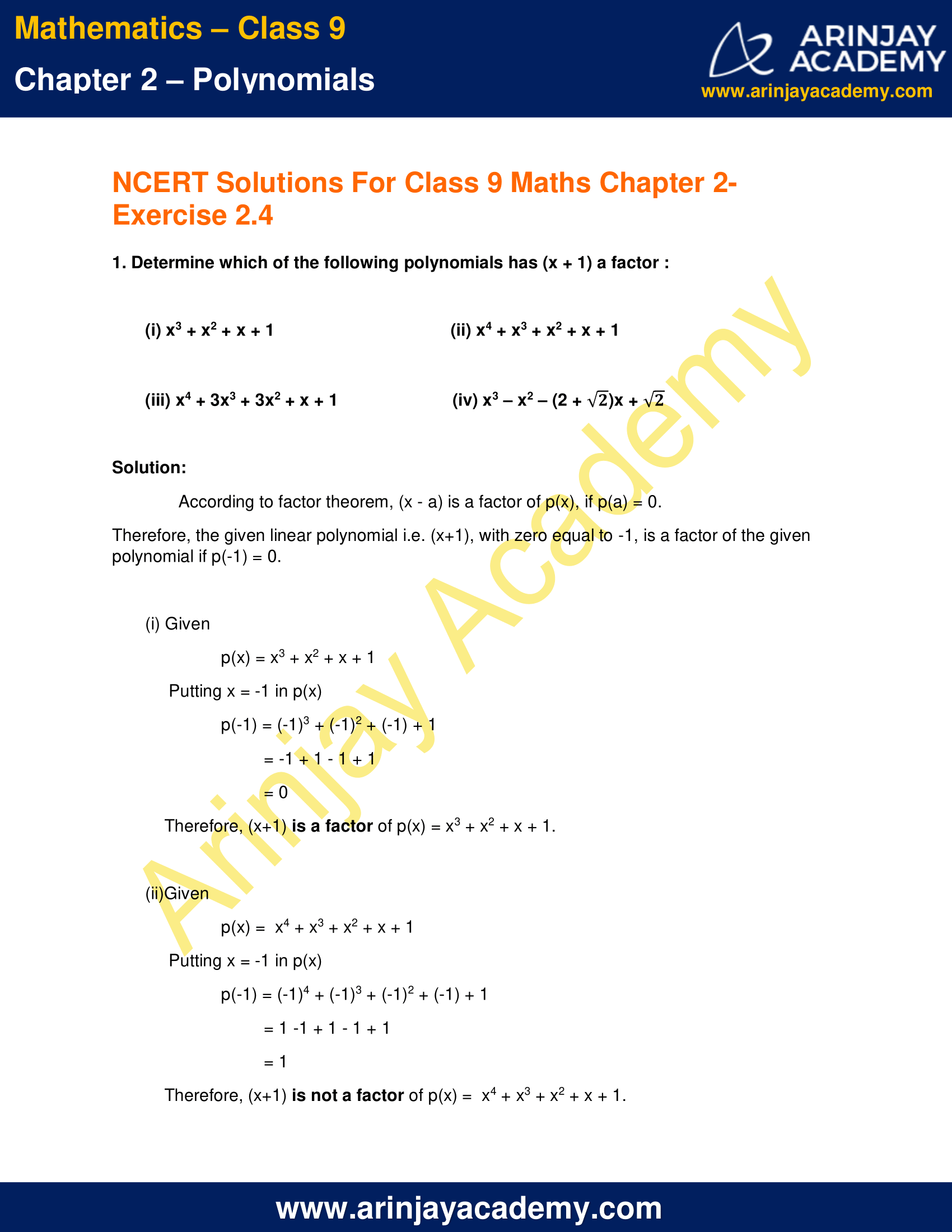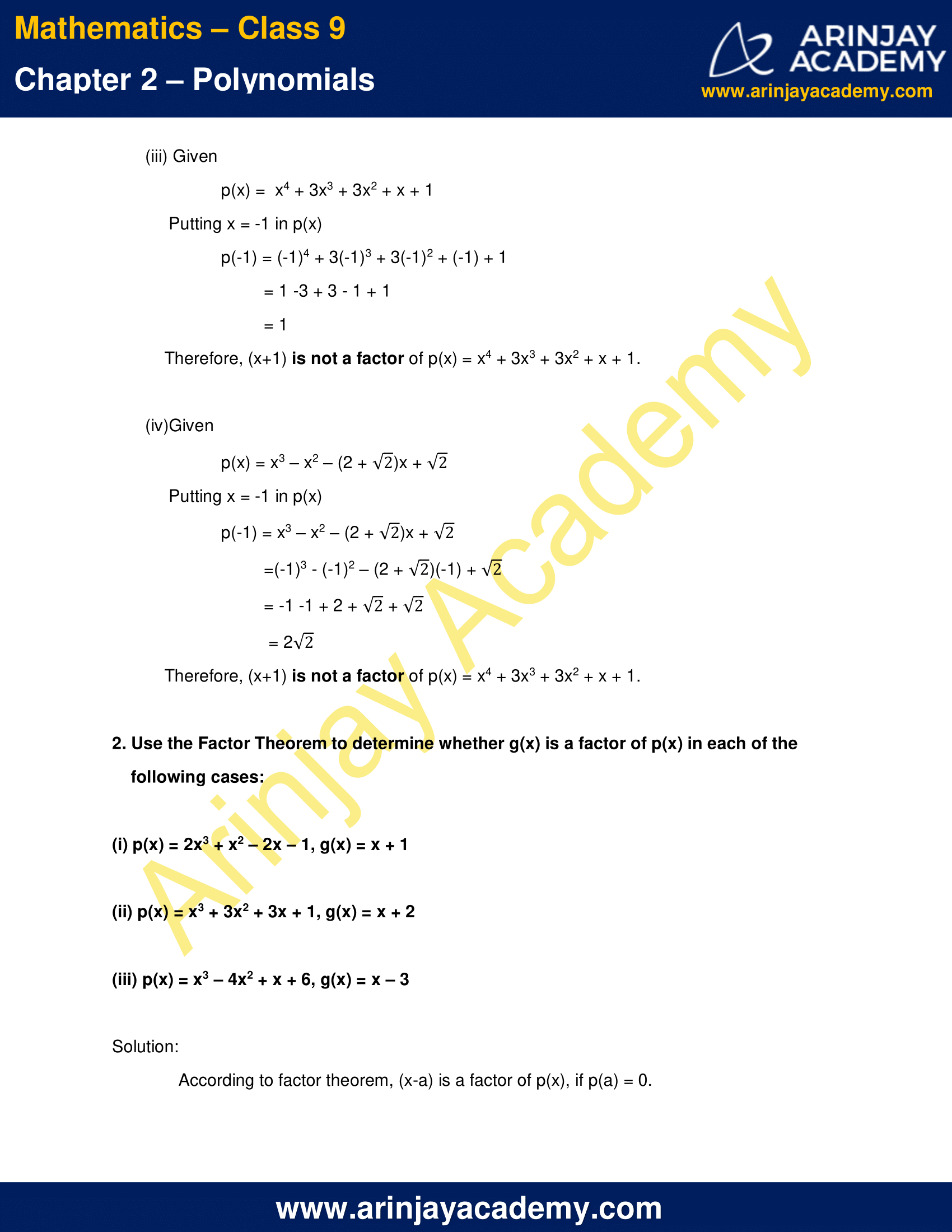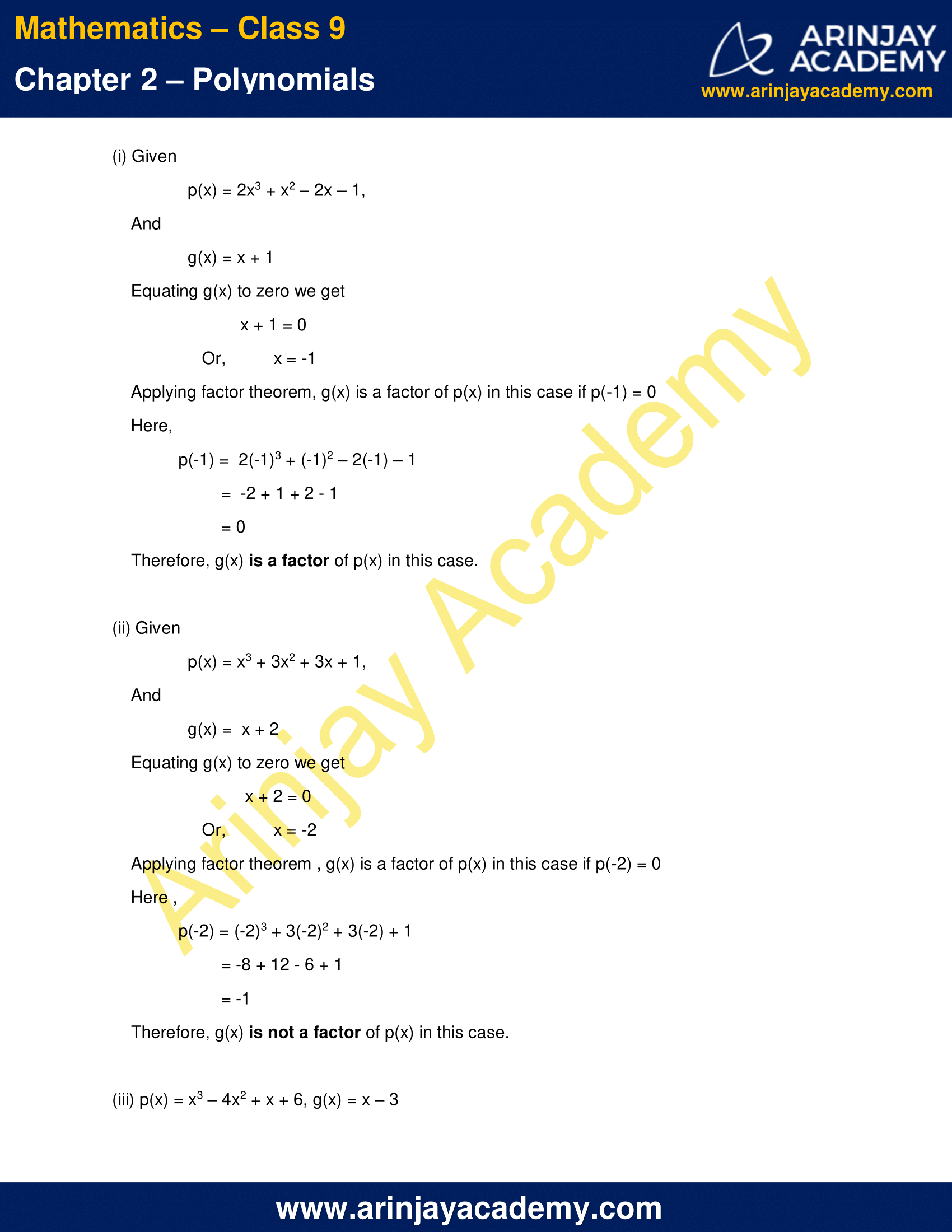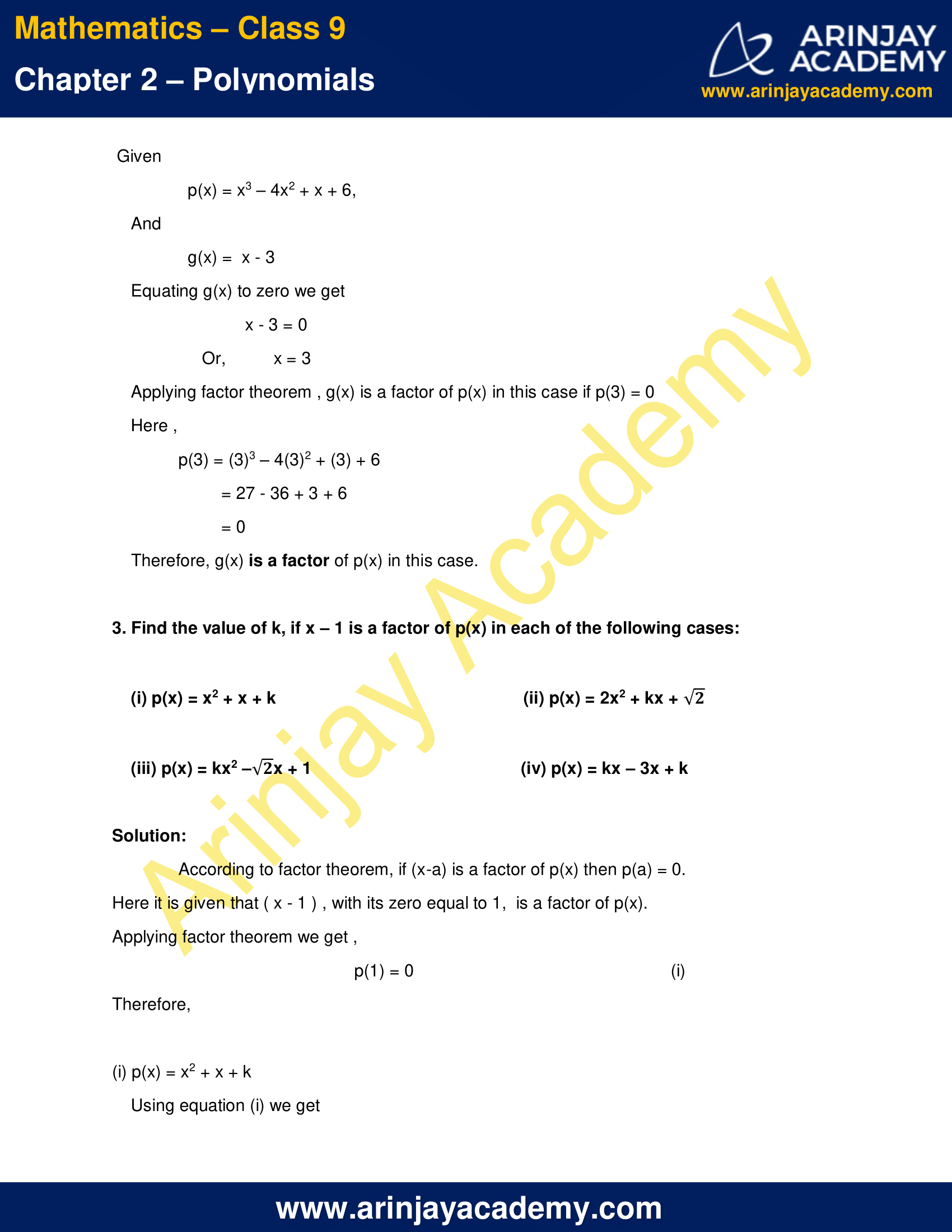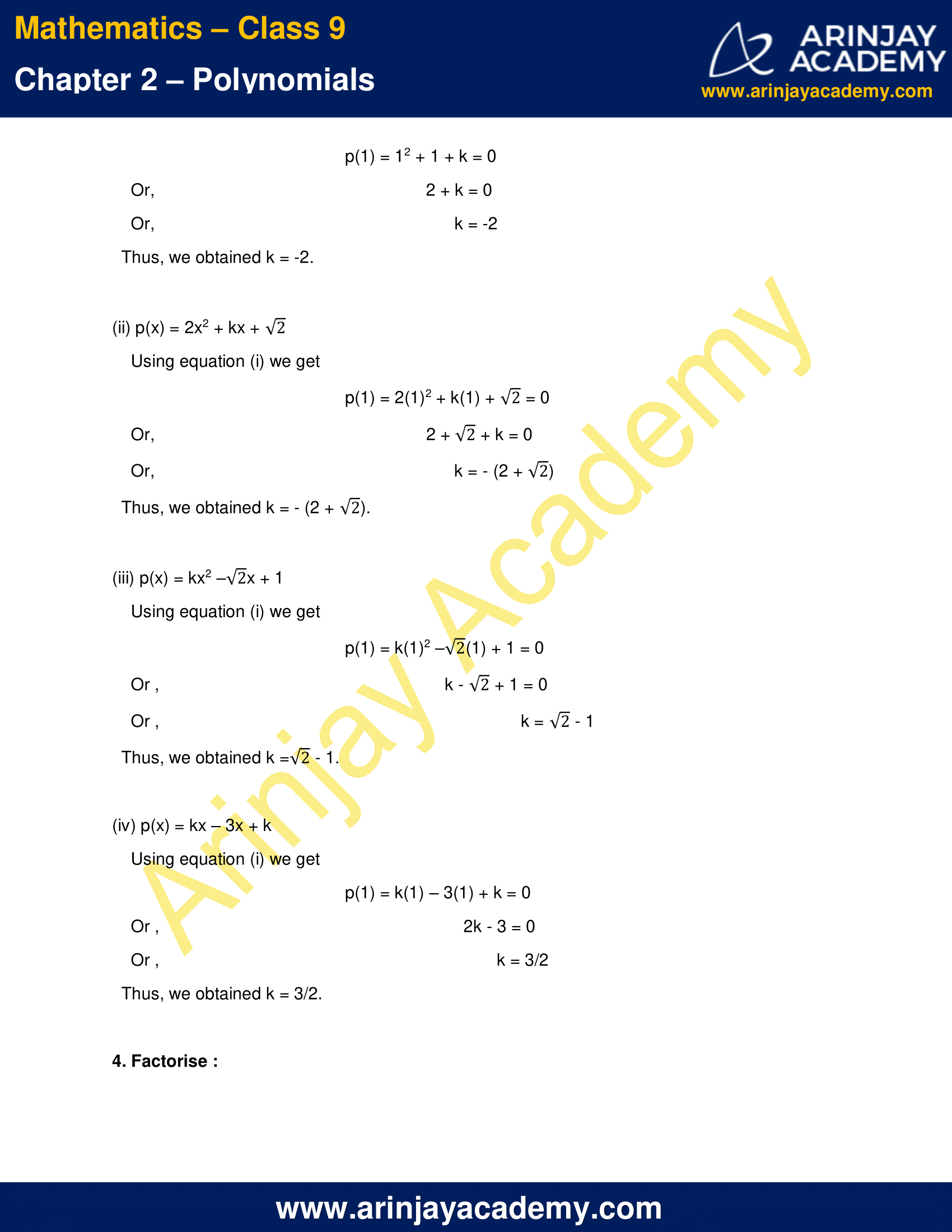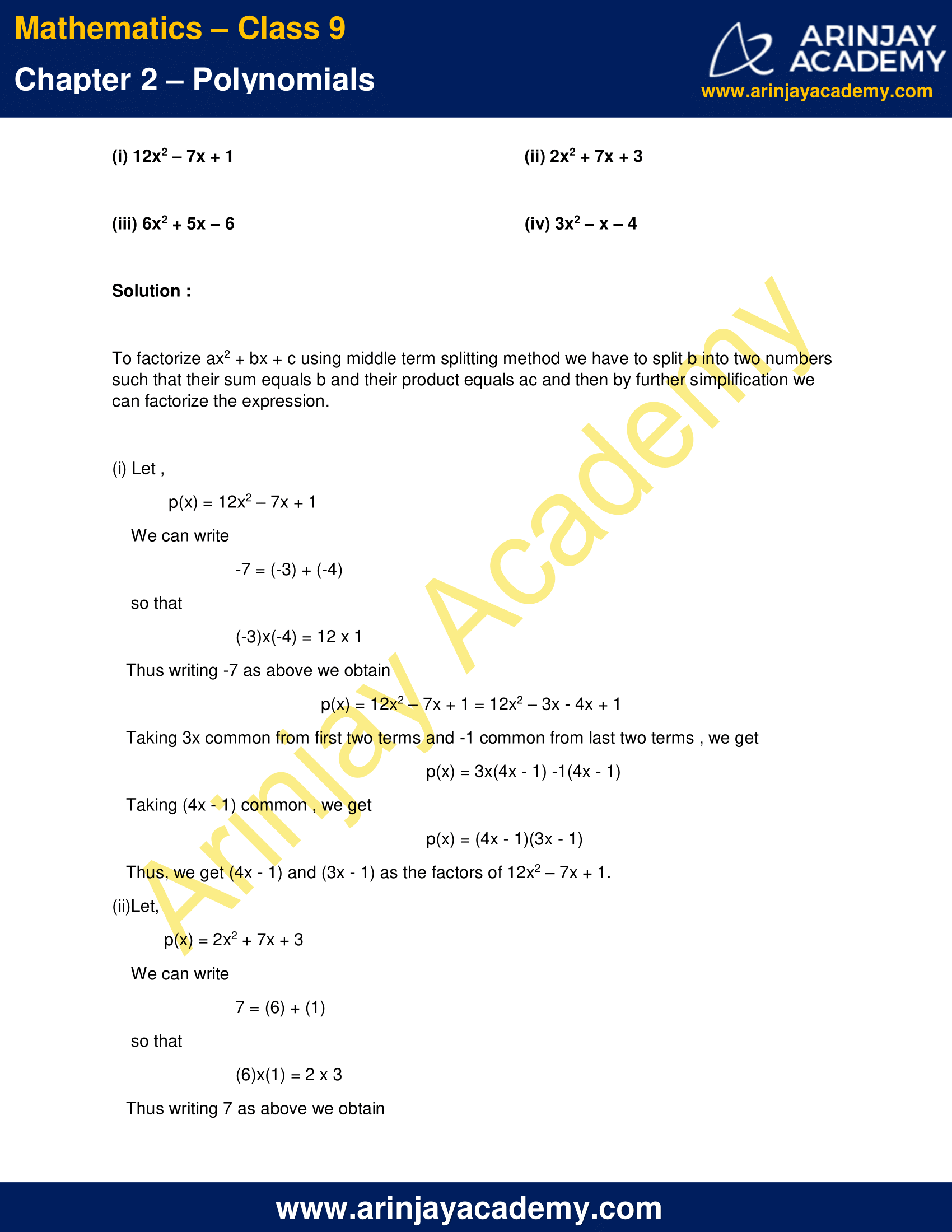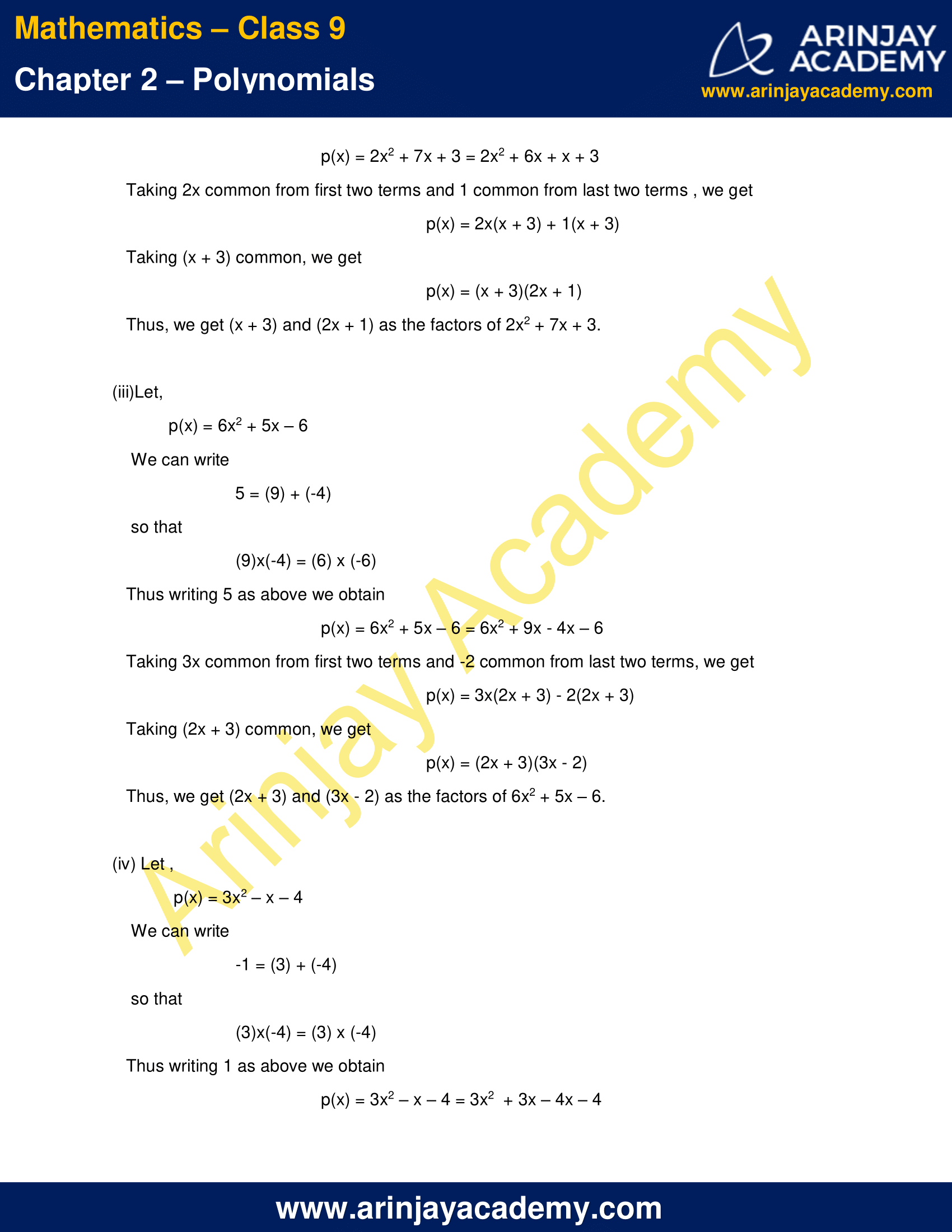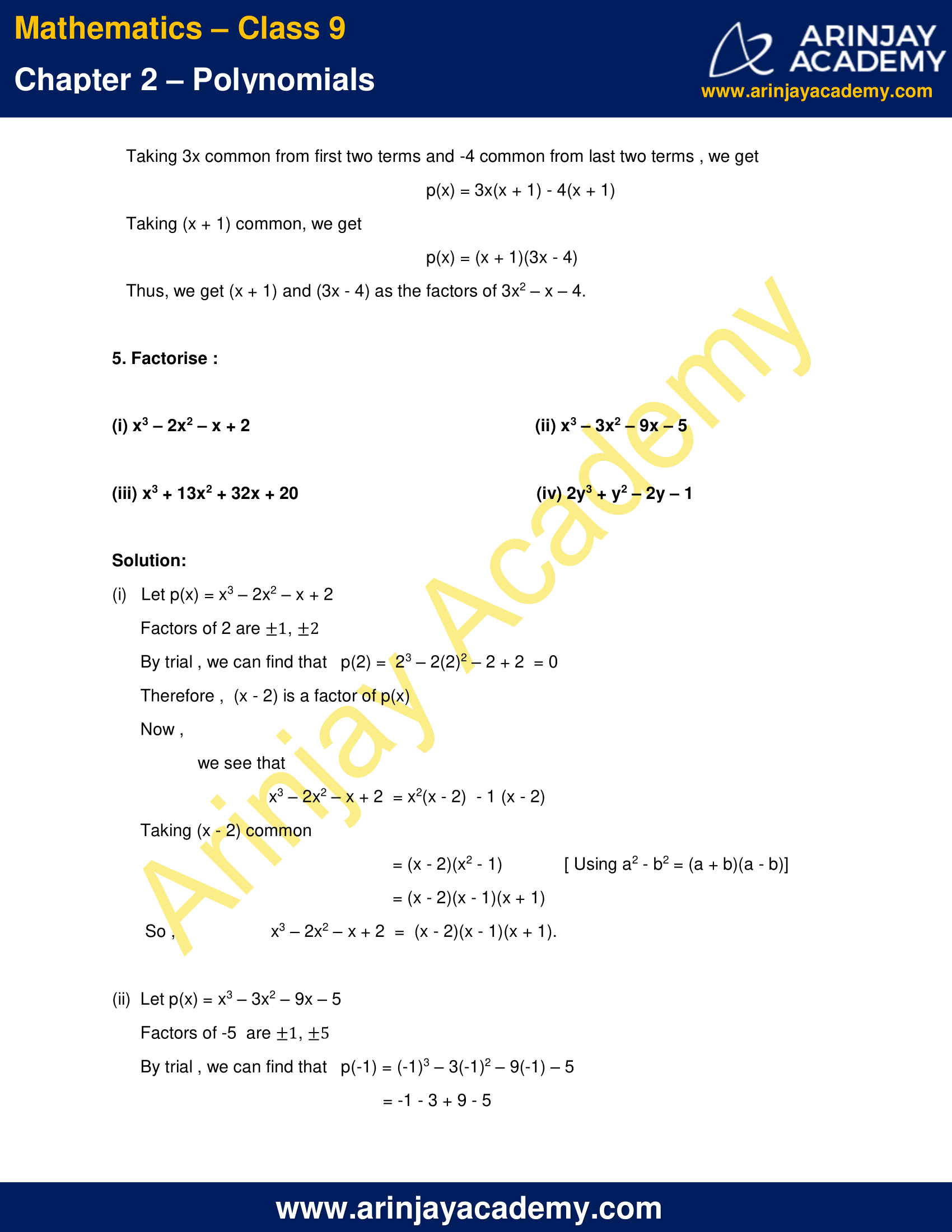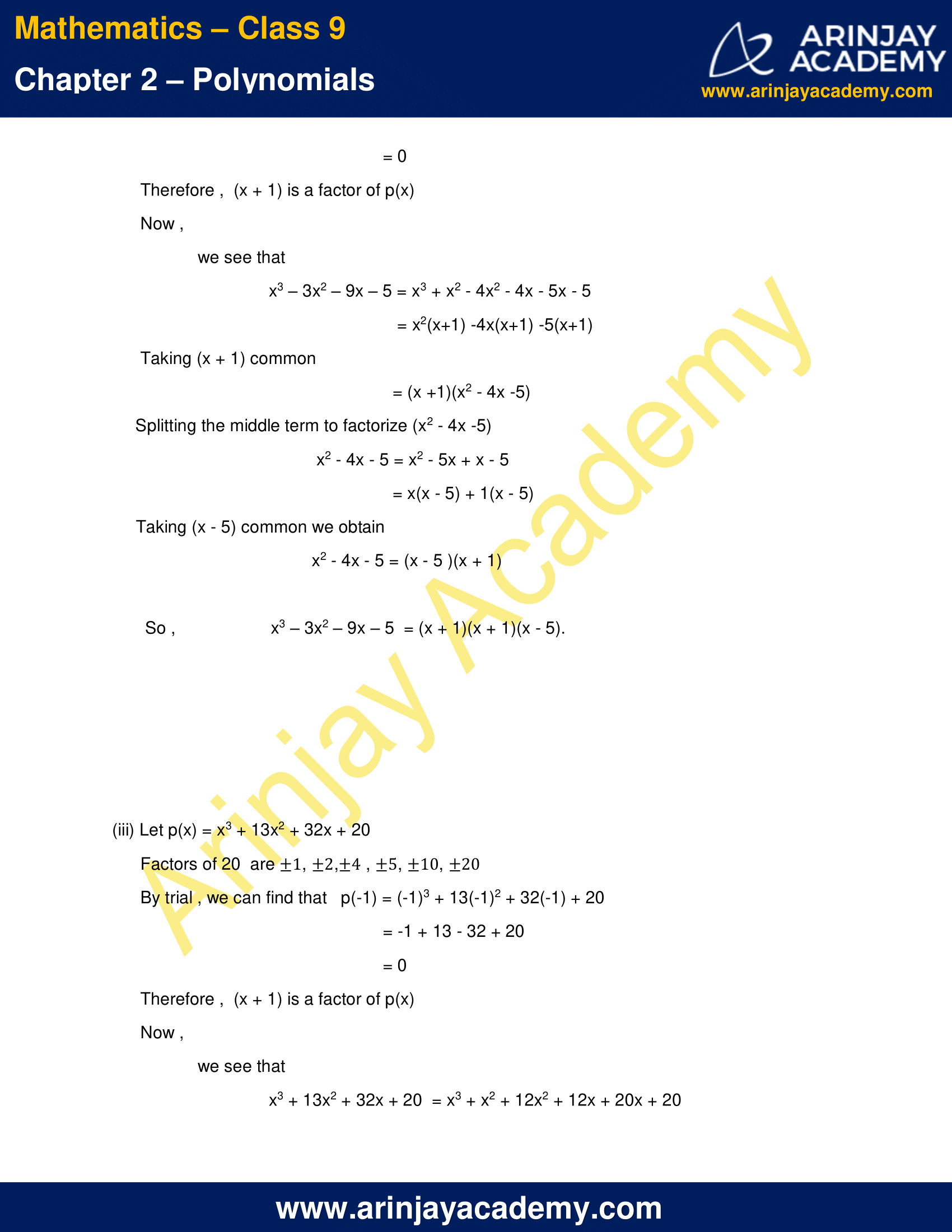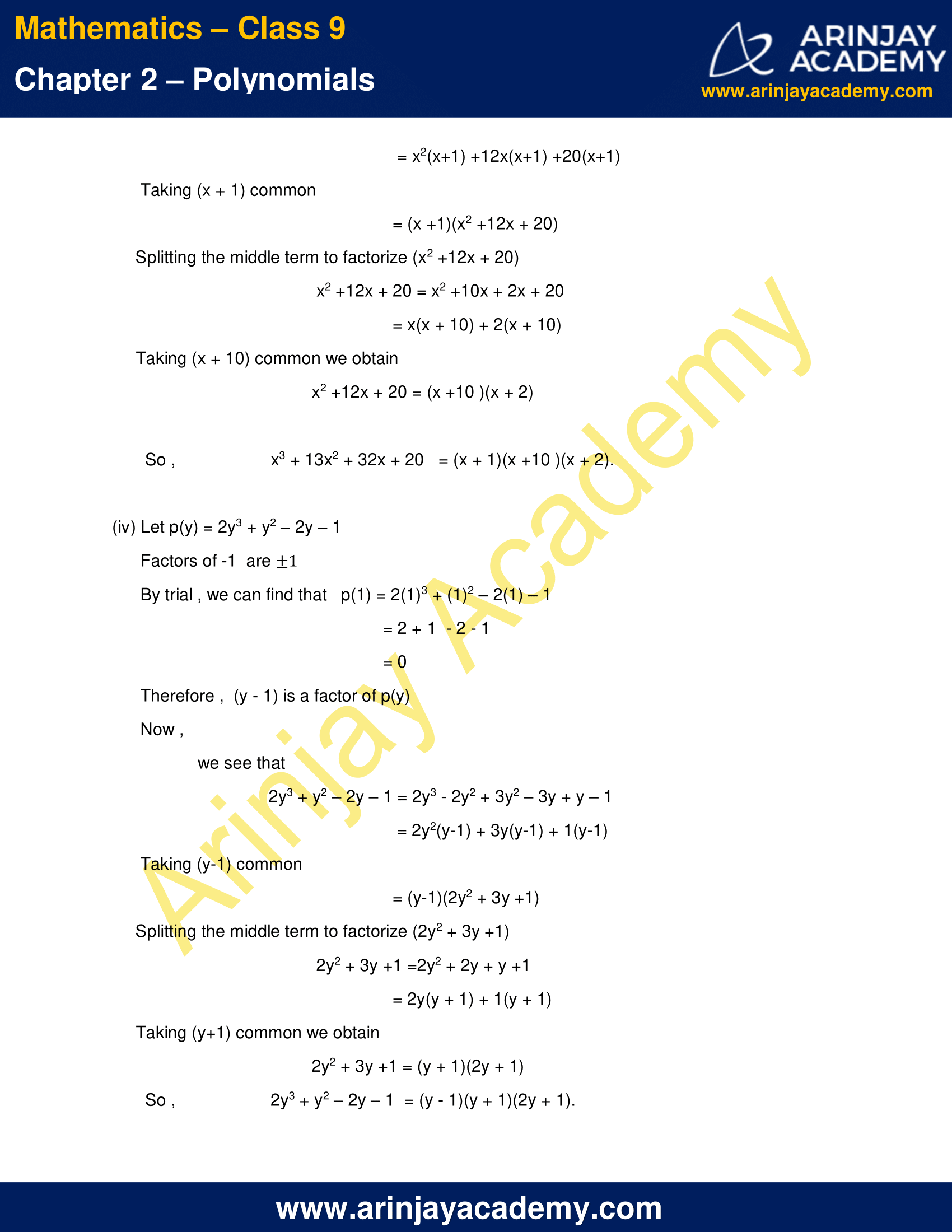NCERT Solutions for Class 9 Maths Chapter 2 Exercise 2.4 – Polynomials

1. Determine which of the following polynomials has (x + 1) a factor :

(i) x3 + x2 + x + 1
(ii) x4 + x3 + x2 + x + 1
(iii) x4 + 3x3 + 3x2 + x + 1
(iv) x3 – x2 – (2 + √2
)x + √2

Solution:

According to factor theorem, (x – a) is a factor of p(x), if p(a) = 0.
Therefore, the given linear polynomial i.e. (x+1), with zero equal to -1, is a factor of the given polynomial if p(-1) = 0.

(i) Given
p(x) = x3 + x2 + x + 1
Putting x = -1 in p(x)
p(-1) = (-1)3 + (-1)2 + (-1) + 1
= -1 + 1 – 1 + 1
= 0
Therefore, (x+1) is a factor of p(x) = x3 + x2 + x + 1.

(ii)Given
p(x) =  x4 + x3 + x2 + x + 1
Putting x = -1 in p(x)
p(-1) = (-1)4 + (-1)3 + (-1)2 + (-1) + 1
= 1 -1 + 1 – 1 + 1
= 1
Therefore, (x+1) is not a factor of p(x) =  x4 + x3 + x2 + x + 1.

(iii) Given
p(x) =  x4 + 3x3 + 3x2 + x + 1
Putting x = -1 in p(x)
p(-1) = (-1)4 + 3(-1)3 + 3(-1)2 + (-1) + 1
= 1 -3 + 3 – 1 + 1
= 1
Therefore, (x+1) is not a factor of p(x) = x4 + 3x3 + 3x2 + x + 1.

(iv)Given
p(x) = x3 – x2 – (2 + √2)x + √2
Putting x = -1 in p(x)
p(-1) = x3 – x2 – (2 + √2)x + √2
=(-1)3 – (-1)2 – (2 + √2)(-1) + √2
= -1 -1 + 2 + √2 + √2
= 2√2
Therefore, (x+1) is not a factor of p(x) = x4 + 3x3 + 3x2 + x + 1.

2. Use the Factor Theorem to determine whether g(x) is a factor of p(x) in each of the following cases:

(i) p(x) = 2x3 + x2 – 2x – 1, g(x) = x + 1
(ii) p(x) = x3 + 3x2 + 3x + 1, g(x) = x + 2
(iii) p(x) = x3 – 4x2 + x + 6, g(x) = x – 3

Solution:

According to factor theorem, (x-a) is a factor of p(x), if p(a) = 0.

(i) Given
p(x) = 2x3 + x2 – 2x – 1,
And
g(x) = x + 1
Equating g(x) to zero we get
x + 1 = 0
Or,          x = -1
Applying factor theorem, g(x) is a factor of p(x) in this case if p(-1) = 0
Here,
p(-1) =  2(-1)3 + (-1)2 – 2(-1) – 1
=  -2 + 1 + 2 – 1
= 0
Therefore, g(x) is a factor of p(x) in this case.

(ii) Given

p(x) = x3 + 3x2 + 3x + 1,
And
g(x) =  x + 2
Equating g(x) to zero we get
x + 2 = 0
Or,          x = -2
Applying factor theorem , g(x) is a factor of p(x) in this case if p(-2) = 0
Here ,
p(-2) = (-2)3 + 3(-2)2 + 3(-2) + 1
= -8 + 12 – 6 + 1
= -1
Therefore, g(x) is not a factor of p(x) in this case.

(iii) p(x) = x3 – 4x2 + x + 6, g(x) = x – 3
Given
p(x) = x3 – 4x2 + x + 6,
And
g(x) =  x – 3
Equating g(x) to zero we get
x – 3 = 0
Or,          x = 3
Applying factor theorem , g(x) is a factor of p(x) in this case if p(3) = 0
Here ,
p(3) = (3)3 – 4(3)2 + (3) + 6
= 27 – 36 + 3 + 6
= 0
Therefore, g(x) is a factor of p(x) in this case.

3. Find the value of k, if x – 1 is a factor of p(x) in each of the following cases:

(i) p(x) = x2 + x + k
(ii) p(x) = 2x2 + kx + √2
(iii) p(x) = kx2 √2x + 1
(iv) p(x) = kx – 3x + k

Solution:

According to factor theorem, if (x-a) is a factor of p(x) then p(a) = 0. Here it is given that ( x – 1 ) , with its zero equal to 1,  is a factor of p(x).
Applying factor theorem we get ,
p(1) = 0 ….(i)
Therefore,

(i) p(x) = x2 + x + k
Using equation (i) we get
p(1) = 12 + 1 + k = 0
Or,      2 + k = 0
Or,      k = -2
Thus, we obtained k = -2.

(ii) p(x) = 2x2 + kx + √2
Using equation (i) we get
p(1) = 2(1)2 + k(1) + √2 = 0
Or,        2 + √2 + k = 0
Or,        k = – (2 + √2 )
Thus, we obtained k = – (2 + √2).

(iii) p(x) = kx2 – √2x + 1
Using equation (i) we get
p(1) = k(1)2 – √2(1) + 1 = 0
Or ,        k – √2 + 1 = 0
Or ,        k = √2 – 1
Thus, we obtained k = √2 – 1.

(iv) p(x) = kx – 3x + k
Using equation (i) we get
p(1) = k(1) – 3(1) + k = 0
Or ,        2k – 3 = 0
Or ,        k = 3/2
Thus, we obtained k = 3/2.

4. Factorise :

(i) 12x2 – 7x + 1
(ii) 2x2 + 7x + 3
(iii) 6x2 + 5x – 6
(iv) 3x2 – x – 4

Solution :

To factorize ax2 + bx + c using middle term splitting method we have to split b into two numbers such that their sum equals b and their product equals ac and then by further simplification we can factorize the expression.

(i) Let ,
p(x) = 12x2 – 7x + 1
We can write
-7 = (-3) + (-4)
so that
(-3)x(-4) = 12 x 1
Thus writing -7 as above we obtain
p(x) = 12x2 – 7x + 1 = 12x2 – 3x – 4x + 1
Taking 3x common from first two terms and -1 common from last two terms , we get
p(x) = 3x(4x – 1) -1(4x – 1)
Taking (4x – 1) common , we get
p(x) = (4x – 1)(3x – 1)
Thus, we get (4x – 1) and (3x – 1) as the factors of 12x2 – 7x + 1.

(ii)Let,
p(x) = 2x2 + 7x + 3
We can write
7 = (6) + (1)
so that
(6)x(1) = 2 x 3
Thus writing 7 as above we obtain
p(x) = 2x2 + 7x + 3 = 2x2 + 6x + x + 3
Taking 2x common from first two terms and 1 common from last two terms , we get
p(x) = 2x(x + 3) + 1(x + 3)
Taking (x + 3) common, we get
p(x) = (x + 3)(2x + 1)
Thus, we get (x + 3) and (2x + 1) as the factors of 2x2 + 7x + 3.

(iii)Let,
p(x) = 6x2 + 5x – 6
We can write
5 = (9) + (-4)
so that
(9)x(-4) = (6) x (-6)
Thus writing 5 as above we obtain
p(x) = 6x2 + 5x – 6 = 6x2 + 9x – 4x – 6
Taking 3x common from first two terms and -2 common from last two terms, we get
p(x) = 3x(2x + 3) – 2(2x + 3)
Taking (2x + 3) common, we get
p(x) = (2x + 3)(3x – 2)
Thus, we get (2x + 3) and (3x – 2) as the factors of 6x2 + 5x – 6.

(iv) Let ,
p(x) = 3x2 – x – 4
We can write
-1 = (3) + (-4)
so that
(3)x(-4) = (3) x (-4)
Thus writing 1 as above we obtain
p(x) = 3x2 – x – 4 = 3x2  + 3x – 4x – 4
Taking 3x common from first two terms and -4 common from last two terms , we get
p(x) = 3x(x + 1) – 4(x + 1)
Taking (x + 1) common, we get
p(x) = (x + 1)(3x – 4)
Thus, we get (x + 1) and (3x – 4) as the factors of 3x2 – x – 4.

5. Factorise :

(i) x3 – 2x2 – x + 2
(ii) x3 – 3x2 – 9x – 5
(iii) x3 + 13x2 + 32x + 20
(iv) 2y3 + y2 – 2y – 1

Solution:

(i)   Let p(x) = x3 – 2x2 – x + 2
Factors of 2 are ±1, ±2
By trial , we can find that   p(2) =  23 – 2(2)2 – 2 + 2  = 0
Therefore ,  (x – 2) is a factor of p(x)
Now ,
we see that
x3 – 2x2 – x + 2  = x2(x – 2)  – 1 (x – 2)
Taking (x – 2) common
= (x – 2)(x2 – 1)             [ Using a2 – b2 = (a + b)(a – b)]
= (x – 2)(x – 1)(x + 1)
So ,  x3 – 2x2 – x + 2  =  (x – 2)(x – 1)(x + 1).

(ii)  Let p(x) = x3 – 3x2 – 9x – 5
Factors of -5  are ±1 , ±5
By trial , we can find that   p(-1) = (-1)3 – 3(-1)2 – 9(-1) – 5
= -1 – 3 + 9 – 5
= 0
Therefore ,  (x + 1) is a factor of p(x)
Now ,
we see that
x3 – 3x2 – 9x – 5 = x3 + x2 – 4x2 – 4x – 5x – 5
= x2(x+1) -4x(x+1) -5(x+1)
Taking (x + 1) common
= (x +1)(x2 – 4x -5)
Splitting the middle term to factorize (x2 – 4x -5)
x2 – 4x – 5 = x2 – 5x + x – 5
= x(x – 5) + 1(x – 5)
Taking (x – 5) common we obtain
x2 – 4x – 5 = (x – 5 )(x + 1)
So,  x3 – 3x2 – 9x – 5  = (x + 1)(x + 1)(x – 5).

(iii) Let p(x) = x3 + 13x2 + 32x + 20
Factors of 20  are ±1, ±2, ±4, ±5, ±10, ±20
By trial , we can find that   p(-1) = (-1)3 + 13(-1)2 + 32(-1) + 20
= -1 + 13 – 32 + 20
= 0
Therefore ,  (x + 1) is a factor of p(x)
Now,
we see that
x3 + 13x2 + 32x + 20  = x3 + x2 + 12x2 + 12x + 20x + 20
= x2(x+1) +12x(x+1) +20(x+1)
Taking (x + 1) common
= (x +1)(x2 +12x + 20)
Splitting the middle term to factorize (x2 +12x + 20)
x2 +12x + 20 = x2 +10x + 2x + 20
= x(x + 10) + 2(x + 10)
Taking (x + 10) common we obtain
x2 +12x + 20 = (x +10 )(x + 2)
So,  x3 + 13x2 + 32x + 20   = (x + 1)(x +10 )(x + 2).

(iv) Let p(y) = 2y3 + y2 – 2y – 1
Factors of -1  are ±1
By trial , we can find that   p(1) = 2(1)3 + (1)2 – 2(1) – 1
= 2 + 1  – 2 – 1
= 0
Therefore ,  (y – 1) is a factor of p(y)
Now ,
we see that
2y3 + y2 – 2y – 1 = 2y3 – 2y2 + 3y2 – 3y + y – 1
= 2y2(y-1) + 3y(y-1) + 1(y-1)
Taking (y-1) common
= (y-1)(2y2 + 3y +1)
Splitting the middle term to factorize (2y2 + 3y +1)
2y2 + 3y +1 =2y2 + 2y + y +1
= 2y(y + 1) + 1(y + 1)
Taking (y+1) common we obtain
2y2 + 3y +1 = (y + 1)(2y + 1)
So, 2y3 + y2 – 2y – 1  = (y – 1)(y + 1)(2y + 1).

NCERT Solutions for Class 9 Maths Chapter 2 Exercise 2.4 – Polynomials, has been designed by the NCERT to test the knowledge of the student on the topic – Factorisation of Polynomials

The next Exercise for NCERT Solutions for Class 9 Maths Chapter 2 Exercise 2.5 – Number System can be accessed by clicking here.

Download NCERT Solutions for Class 9 Maths Chapter 2 Exercise 2.4 – Polynomials